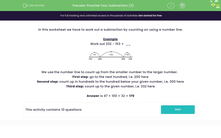### Comprehensive & curriculum aligned

In this worksheet, students work out a subtraction by counting using a number line.Key stage:  KS 2

Curriculum topic:   Number: Addition and Subtraction

Curriculum subtopic:   Solve Add/Subtract Problems to 1000

Difficulty level:#### Worksheet Overview

In this worksheet we have to work out a subtraction by counting on using a number line.

Example

Work out 332 - 153 =  __We use the number line to count up from the smaller number to the larger number.

First step: go to the next hundred, i.e. 200 here

Second step: count up in hundreds to the hundred below your given number, i.e. 300 here

Third step: count up to the given number, i.e. 332 here

Answer is 47 + 100 + 32 = 179

### What is EdPlace?

We're your National Curriculum aligned online education content provider helping each child succeed in English, maths and science from year 1 to GCSE. With an EdPlace account you’ll be able to track and measure progress, helping each child achieve their best. We build confidence and attainment by personalising each child’s learning at a level that suits them.

Get started